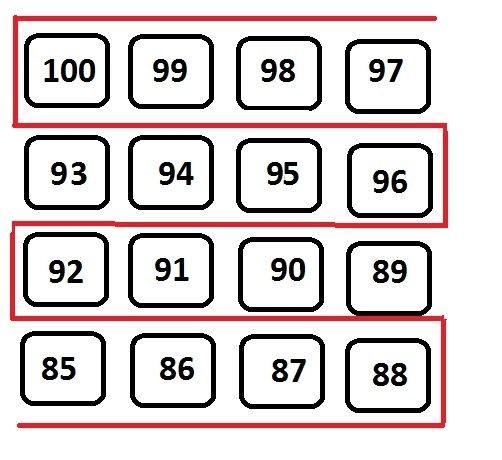# Print matrix in snake pattern from the last column in C Programming.

CServer Side ProgrammingProgramming

#### C in Depth: The Complete C Programming Guide for Beginners

45 Lectures 4.5 hours

#### Practical C++: Learn C++ Basics Step by Step

Most Popular

50 Lectures 4.5 hours

#### Master C and Embedded C Programming- Learn as you go

66 Lectures 5.5 hours

Given an array of nxn size, the program must print the elements of an array in a snake pattern starting from the last column that means from arr[n]th element without doing any changes to their original locations.## Example

Input: arr[]= 100 99 98 97
93 94 95 96
92 91 90 89
85 86 87 88
Output: 97 98 99 100 96 95 94 93 92 91 90 89 88 87 86 85

## Algorithm

START
Step 1 -> declare initial variable as int n to 5, i and j
Step 2 -> declare array of 2-D matrix with elements
Step 3 -> Loop For i=0 and i<n and i++
IF i%2==1
Loop For j=0 and j<n and j++
Print arr[i][j]
End
End
Else
Loop For j=n-1 and j>=0 and j--
Print arr[i][j]
End
End
STOP

## Example

Live Demo

#include <stdio.h>
int main(){
int n = 5;
int arr[]= {
{10, 20, 30, 40, 50},
{ 60, 70, 80, 90, 100 },
{ 110, 120, 130, 140, 150 },
{ 160, 170, 180, 190, 200 },
{ 210, 220, 230, 240, 250 }
};
for (int i = 0; i < n; i++) { //from column as we must start from the end
if (i%2 == 1)
for (int j = 0; j < n; j++)
printf("%d ", arr[i][j]);
else
for (int j = n - 1; j >= 0; j--)
printf("%d ", arr[i][j]);
}
return 0;
}

## Output

if we run the above program then it will generate the following output

50 40 30 20 10 60 70 80 90 100 150 140 130 120 110 160 170 180 190 200 250 240 230 220 210